Community /

# The Ultimate Guide for FFT Analysis in OpenBCI GUI and Matlab

This is the ULTIMATE GUIDE for FFT in OpenBCI and Matlab!!
You may wonder what does FFT mean, you may want to use some of its value in the GUI but don’t know where and how, you may want to reimplement the good-looking FFT from the GUI to Matlab for future use…
From intro level to advanced, hopefully you will find all you need here 🙂

Lv1 (beginner): Understanding the FFT Plot
The quests below are the goals of this section. If you can answer all of them, you can skip this section and move on to the next.
//——————-  begin quests #1 ——————–
If alpha band is defined as a frequency band between 7 – 13Hz, you can see a peak in this band in the below image. Then what is the frequency and amplitude of this peak? What does it mean?
Quest 1-2: Sampling rate and time span
If the length of FFT is 256, and sampling rate is 250Hz. How many milliseconds of data does it compute?
Quest 1-3: Center frequency and index of a bin
If the length of FFT is 256, and sampling rate is 250Hz. If the index of bins starts from 0, then what is the center frequency of 25th bin? What is the index of the bin that 20Hz belongs to?
Quest 1-4: Window function
What’s the benefit of using a window function?
Quest 1-5: Buffer refreshing and smooth factor
How fast does the FFT buffer typically refresh itself? If the default value of FFT smooth factor in the GUI is 0.75, and you want the FFT Plot to be even smoother in time, should you choose a higher or lower value?

//——————– end of quests #1 ——————–

It is known that brainwaves displays a couple of rhythmic behaviors at times. For example, when a healthy people closes their eyes, it is likely their brainwaves will show a rhythmic pattern that vibrates 7-13 times a second, and this pattern is named alpha wave. Since this feature is frequency related, FFT can be used to easily show the frequency components, the aforementioned alpha activity for example.
Alpha activity (you can count around 10 peaks between two adjacent bars, i.e. 10 cycles in 1 second)

What is FFT?
Fast Fourier Transform (FFT) is a very powerful tool for revealing the useful frequency components of a signal, even when the signal is influenced by noise. FFT originates from the Fourier Transform (FT), a mathematical way to extract the frequency information by decomposing any periodic signal into a combination of sine and cosine waves. To adapt to digital signals, a discrete version of Fourier Transform is proposed and named Discrete Fourier Transform (DFT). To compute DFT more efficiently, a faster algorithm was developed — the FFT.
Starting from FT, below is an animation (from wikipedia) that illustrates how it works. You can see a square wave signal is decomposed into sine and cosine waves of different frequencies (frequency components), and each frequency component has a specific amplitude (and phase, though we won’t elaborate on this). The blue graph in the animation is called an amplitude spectrum. It shows the amplitudes at different frequencies , where the horizontal axis means frequency, and the vertical axis means amplitude.In practice, since the computer cannot process a continuous signal, the original signal is sampled into a group of discrete data points at specific sampling times. The speed of sampling is called sampling rate. For example, most of our boards use a sampling rate at 250Hz, it means 250 data points will be required in a second, and the interval between two adjacent data points will be 1/250 s = 4 ms. The sampling process is shown below.
As the original data being discrete, the output of FFT will also be a set of discrete data points as shown in the image below. If FFT takes in L sampled data points (named length-L FFT) at a sampling rate of Fs, it then yields L data points or L frequency bins, where the i-th bin (i starts from 0) has a center frequency of i*Fs/L and is as wide as Fs/L in the spectrum. Usually L is a power of two. Since the raw FFT amplitude spectrum is symmetrical, it is then folded into single-sided spectrum to reveal the true amplitudes. The new spectrum will have a frequency range from 0 ~ Fs/2, starting from the center of 0-th to the L/2-th bin, covering L/2+1 bins in total.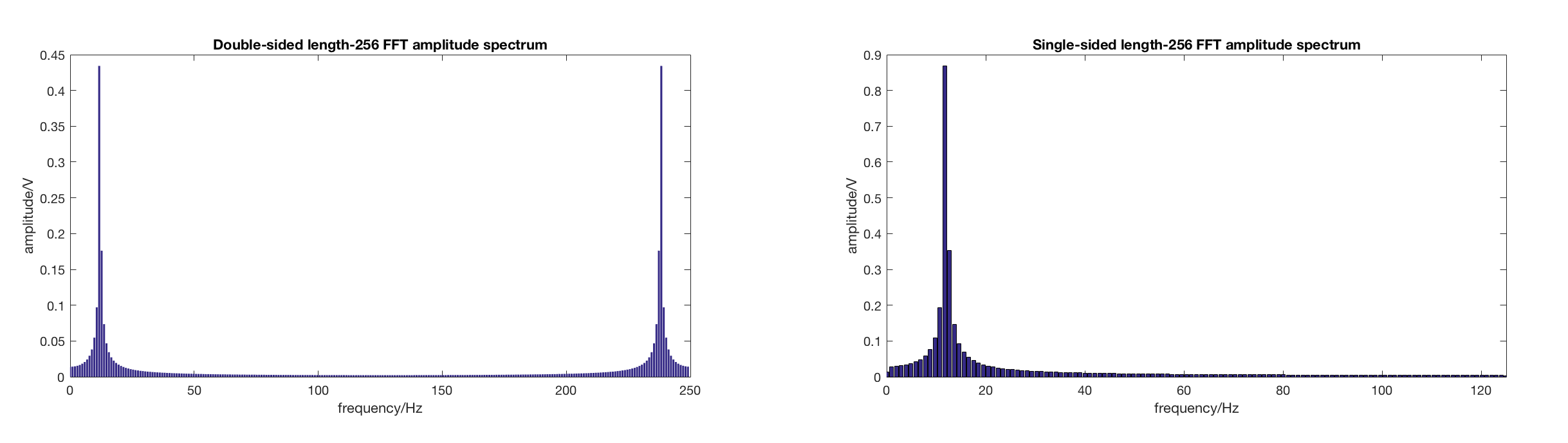How to read the FFT plot in the OpenBCI GUI?
In the GUI, the FFT plot we see is basically the FFT amplitude spectrum of processed signal (explained later in advanced section), and the result of FFT is smoothed over time in dB space.
Then, how to read a given amplitude spectrum? For example, if you see a given FFT amplitude spectrum like the picture below, you can see a peak at around 12 Hz for around 0.87 uV. This means that the original signal is primarily composed of a sine wave that vibrates at around 12Hz and between -0.87V to +0.87V.
In fact, the original signal is a sine wave with 1.0V amplitude and 12Hz frequency. This difference is caused when the sampled data are not representative enough, then FFT will assume smaller amplitudes spreading around the actual frequency in the spectrum, and lowing the peak amplitude. This phenomenon is called spectral leakage.
To handle spectral leakage, window functions are introduced. The image below shows the ideal Fourier transform, FFT without windowing, and FFT after applying a hamming window. You can see that though peak amplitude is attenuated even more, the spectral leakage is reduced after windowing. (Better if applying energy compensation)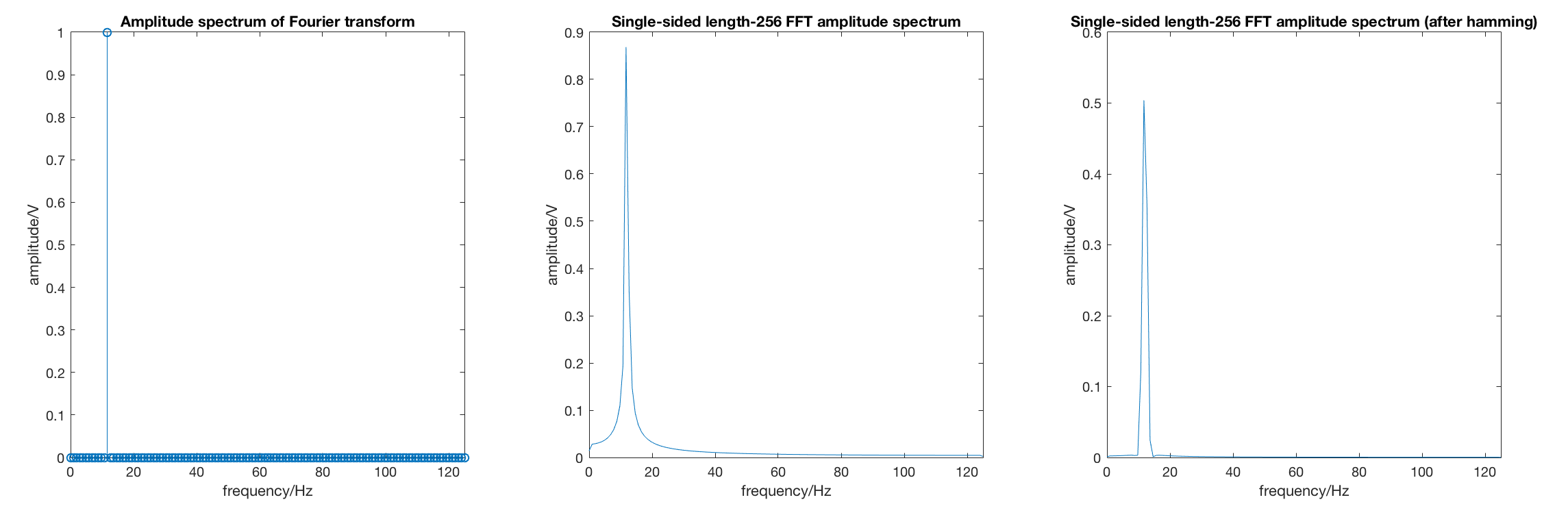Smoothed over time?
As the EEG data buff usually refreshes every 1/5 second, the FFT calculates the latest dataset every 1/5 second as well. FFT values are then smoothed in dB space between the current FFT values and the last FFT values. A smooth factor between 0-1 is then used. The factor means the weight of a previous set of FFT values, thus the higher the factor is, the smoother the FFT will be.
Keys:
1-1 The frequency of this peak is around 10Hz, and it’s amplitude is around 2 uV.
1-2 Because sampling rate means how many data points in a second is required. In this case, the time span between 2 adjacent data points is 1/250 s. And length-256 FFT computes 256 data points. Therefore, the timespan of 256 data points is 256 * 1/250 s = 1.024 s = 1024 ms.
1-3 Since the 0Hz is the center frequency of 0-th bin, and 250 Hz is the center frequency of 256-th bin, the center frequency of the 25th bin will be 25*250/256 = 24.41 Hz. Since the possible index of 20 Hz is 20*256/250 = 20.48, it should belong to the 20th bin. (The center frequency of 20th bin is 19.53, the center frequency of 21th bin is 20.5, the border between the two bins is 19.53+20.5 = 20.015Hz, so 20Hz falls in the right half of the 20th bin).
1-4 decreasing the effect of spectral leakage.
1-5 It typically refreshes every 1/5 second. To make the plot smoother a higher smooth factor, e.g. 0.9, is desired.

Lv2 (Intermediate): Play with FFT inside the GUI
The quests below are the goals of this section. If you can answer all of them, you can skip this section and move on to the next.
//——————-  begin quests #2 ——————–
Quest 2-1: starting point
A convenient place in the GUI to your own FFT code?
Quest 2-2: alpha peak
How to write a piece of code to find the peak frequency (frequency of peak) in alpha band, if alpha band is defined as 7-13 Hz?
Quest 2-3: information flow
What processes are passed from original EEG data to the final smoothed FFT ?
//——————-  end of quests #2 ——————–
Setting-up code in the GUI: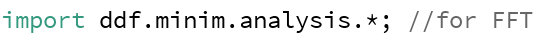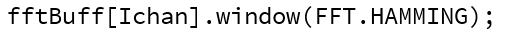OpenBCI GUI uses Minim library to calculate FFT.
Nfft registers the length of FFT, and fftBuff[] holds the most recent FFT data from each channel.
The sampling rate of OpenBCI board can be accessed by calling openBCI.get_fs_Hz(). This sampling rate is asked by Minim to convert bin index into center frequency.
A Hamming window is then specified to FFT calculation (to reduce spectral leakage).
If you want to creating something fun with FFT, your starting point will be this trunk of code below. You will be able to find it by searching FFT_freq_Hz after checking “All Tabs” in the OpenBCI GUI.
The outer loop goes through all available channels, and the inner loop goes through all valid FFT bins.
fftData (same as fftBuff) holds the single-sided amplitude spectrum of nchan channels of data, and each channel has L/2+1 FFT bins. This number of bins can be accessed by .specSize() function provided by Minim.
Then inside the loop, those two are the values you will need:
fftData[Ichan].indexToFreq(Ibin) is a function in the Minim library. It returns the center frequency of the FFT bin (x value in FFT plot) by looking up its index.
fftData[Ichan].getBand(Ibin) is a function in the Minim library. It returns the amplitude of the FFT bin in uV.
Keys:
2-1 Download your copy of the OpenBCI GUI. Open Edit->Find, check “All tabs”, type in “FFT_freq_Hz”. You should be able to find the needed piece of code.
2-2 A sample piece of code (green lines are the added lines):
2-3 The most recent 256 points of raw EEG data is passed inside EEG buff, a hamming window is used before calculation, then single-sided FFT spectrums are calculated and stored inside fftBuff[], then fftBuff[] values are smoothed with previous fftBuff[] values.
sampling raw_uV -> Hamming window -> fftBuff[] -> smoothed fftBuff[]

No quiz here. Read if you like 🙂
The whole story about FFT inside the GUI:
Import Minim library, initialize FFT:Calculating FFT & converting to single-band:

Smoothing in dB space: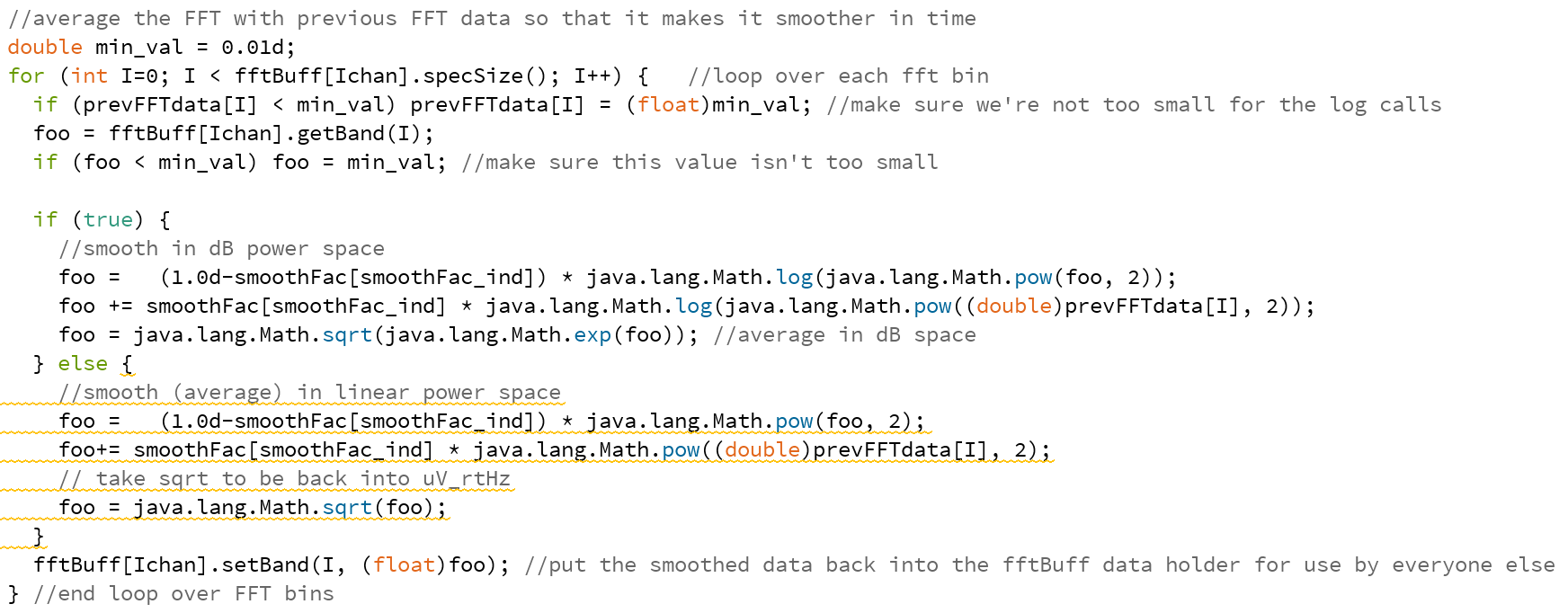Matlab version that replicates GUI processes
Overview:
(The older version of GUI calculates FFT from raw data, the newer version will apply FFT to filtered data. Here use filtered EEG)
Windowing and calculating single-sided FFT amplitude spectrum:
1. The interval is defined as how fast buffer refresh itself. Here 50 data points under 250Hz sampling rate means 1/5 second.
2. In order to reduce the spectral leakage, apply a hamming window to the original signal: temp_han = temp_dat’.*hamming(L);
3. Then, the equation to calculate FFT amplitude is: temp_mag = abs(fft(temp_han));
4. In order to convert it to single-sided spectrum, throw the right half of the FFT spectrum, and then multiply any point except the first (DC) and the last (at Nyquist frequency) by two to correct the amplitude: temp_mag = temp_mag(1:L/2+1); temp_mag(2:end-1) = 2*temp_mag(2:end-1);
Smoothing in dB space: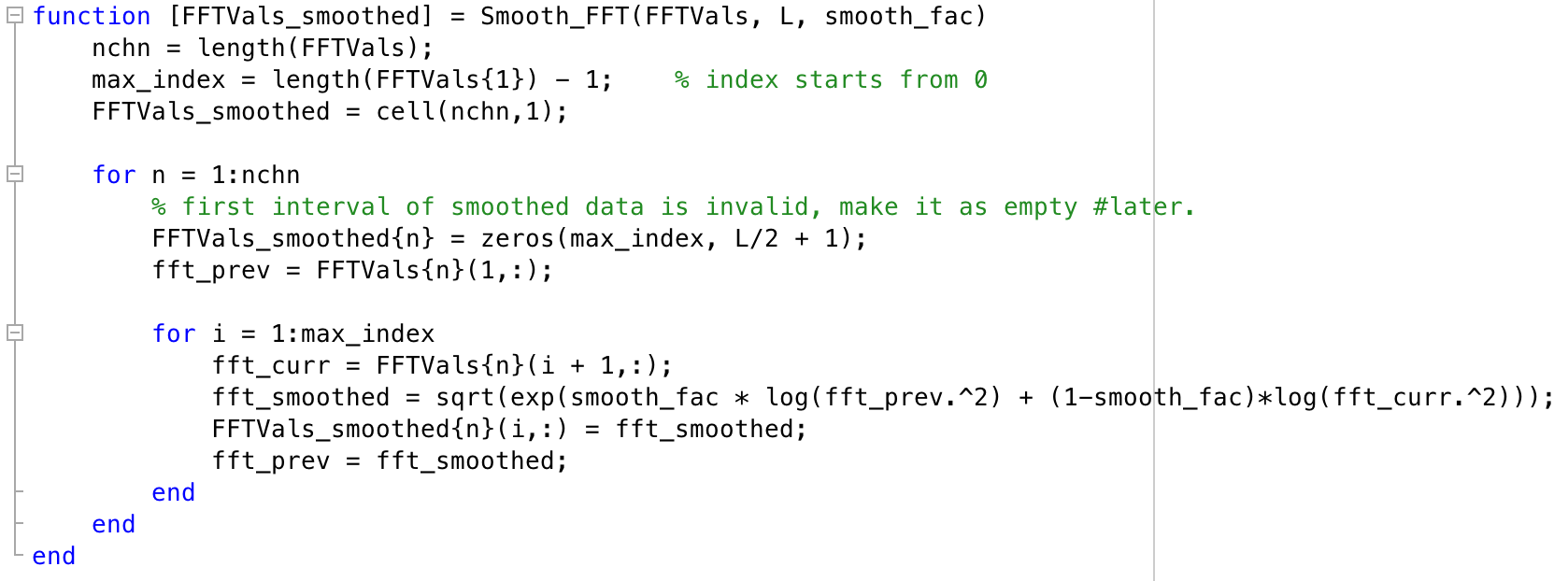The above Matlab code will be available soon.